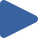# Quick Sort 3 Way

## Animation, code, analysis, and discussion of quick sort (3 way partition) on 4 initial conditions.

How to use: Press "Play all", or choose thebutton.

 Play All Play animationRandomPlay animationNearly SortedPlay animationReversedPlay animationFew Unique### ALGORITHM

``````_# choose pivot_
swap a[n,rand(1,n)]

_# 3-way partition_
i = 1, k = 1, p = n
while i < p,
if a[i] < a[n], swap a[i++,k++]
else if a[i] == a[n], swap a[i,--p]
else i++
end
_→ invariant: a[p..n] all equal_
_→ invariant: a[1..k-1] < a[p..n] < a[k..p-1]_

_# move pivots to center_
m = min(p-k,n-p+1)
swap a[k..k+m-1,n-m+1..n]

_# recursive sorts_
sort a[1..k-1]
sort a[n-p+k+1,n]
``````

### DISCUSSION

The 3-way partition variation of quick sort has slightly higher overhead compared to the standard 2-way partition version. Both have the same best, typical, and worst case time bounds, but this version is highly adaptive in the very common case of sorting with few unique keys.

The 3-way partitioning code shown above is written for clarity rather than optimal performance; it exhibits poor locality, and performs more swaps than necessary. A more efficient but more elaborate 3-way partitioning method is given in Quicksort is Optimal by Robert Sedgewick and Jon Bentley.

When stability is not required, quick sort is the general purpose sorting algorithm of choice. Recently, a novel dual-pivot variant of 3-way partitioning has been discovered that beats the single-pivot 3-way partitioning method both in theory and in practice.

### KEY

• Black values are sorted.
• Gray values are unsorted.
• Dark gray values denote the current interval.
• A pair of red triangles mark k and p (see the code).

### PROPERTIES

• Not stable
• O(lg(n)) extra space
• O(n2) time, but typically O(n·lg(n)) time
• Adaptive: O(n) time when O(1) unique keys